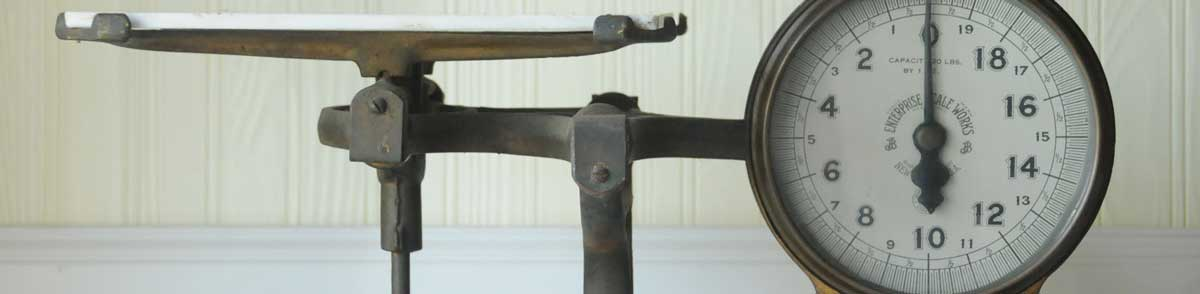# Quarts to Ounces Conversion Calculator

Enter the volume in quarts below to calculate the weight in ounces.

Results in Ounces:1 qt = 33.381617808077 oz
Do you want to convert ounces to quarts?

## How to Convert Quarts to OuncesTo convert a quart measurement to an ounce measurement, multiply the volume by 33.381618 times the density of the ingredient or material.

You can use this simple formula to convert:

ounces = quarts × 33.381618 × ingredient density

Thus, the volume in ounces is equal to the quarts multiplied by 33.381618 times the density of the ingredient or material.

For example, here's how to convert 5 quarts to ounces for an ingredient with a density of 0.7 g/mL.
5 qt = 5 × 33.381618 × 0.7 = 116.835662 oz

This calculator converts to ounces in weight, if you're trying to convert to a volume measurement in fluid ounces try our quarts to fluid ounces calculator.

Quarts and ounces are both commonly used to measure cooking ingredients.

For cooking applications, most chefs suggest measuring dry ingredients by weight rather than volume to improve accuracy in the measurements. The density of dry ingredients can vary for a variety of reasons, such as compaction.

The best way to ensure an accurate conversion is to use a scale. When a scale is not available, a calculator like the one above is a good way to estimate the volume to weight conversion.

## How Many Ounces are in a Quart?

The actual weight of a quart will vary depending on the density of the material. The table below shows how many ounces of various wet and dry ingredients are in a quart.

Quart measurements converted to ounces for commonly used ingredients.
Volume in Quarts: Weight in Ounces of:
Water Milk Cooking Oil All Purpose Flour Granulated Sugar
1/8 qt 4.1727 oz 4.3396 oz 3.672 oz 2.2074 oz 2.9209 oz
1/4 qt 8.3454 oz 8.6792 oz 7.344 oz 4.4147 oz 5.8418 oz
1/3 qt 11.13 oz 11.57 oz 9.7919 oz 5.8863 oz 7.789 oz
1/2 qt 16.69 oz 17.36 oz 14.69 oz 8.8294 oz 11.68 oz
2/3 qt 22.25 oz 23.14 oz 19.58 oz 11.77 oz 15.58 oz
3/4 qt 25.04 oz 26.04 oz 22.03 oz 13.24 oz 17.53 oz
1 qt 33.38 oz 34.72 oz 29.38 oz 17.66 oz 23.37 oz

## Quarts

The US liquid quart is a unit of fluid volume equal to one fourth of a gallon, two pints, or four cups. The liquid quart should not be confused with the dry quart (US) or the imperial quart, which are different units.

The quart is a US customary unit of volume. Quarts can be abbreviated as qt; for example, 1 quart can be written as 1 qt.

## Ounces

One ounce is a unit of mass/weight equal to 1/16 of a pound. The common ounce should not be confused with the troy ounce, which is equal to 1/12 of a troy pound.

The ounce is a US customary and imperial unit of weight. Ounces can be abbreviated as oz; for example, 1 ounce can be written as 1 oz.

## References

1. National Institute of Standards & Technology, Metric Cooking Resources, https://www.nist.gov/pml/weights-and-measures/metric-cooking-resources
2. James Bucki, Troy Ounce vs. Ounce, The Spruce, https://www.thesprucecrafts.com/troy-ounce-vs-ounce-4588158